# Precalculus

Log in to track progressTake the next step in Algebra! Here we'll introduce the most common functions you're likely to see, and different ways to describe functions.

« Back to dashboard
1. Real numbers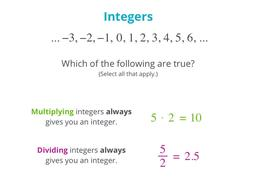Integers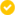Whole numbers, positives, and negatives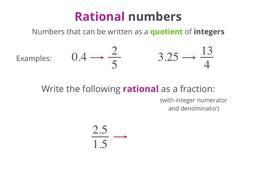RationalsNumbers that are quotients of integers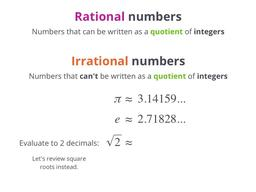IrrationalsNumbers that are NOT quotients of integers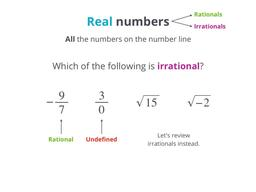Real numbersAll the rationals and the irrationals
2. Imaginary and complex numbers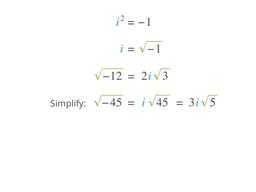Imaginary numbersTheir squares are negative!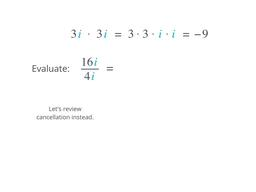Working with imaginariesAdd, subtract, multiply, and divide imaginary numbers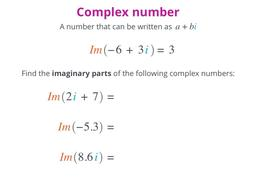Complex numbersCombining the real and the imaginary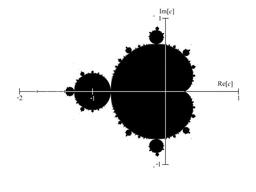The complex planeA way to graph complex numbers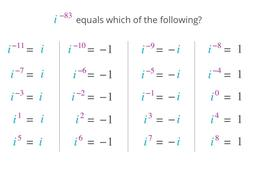Powers of iRaising i to positive and negative integer powers
3. Functions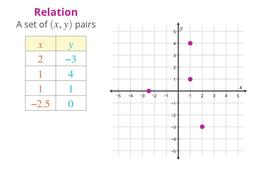RelationsSets of (x,y) coordinates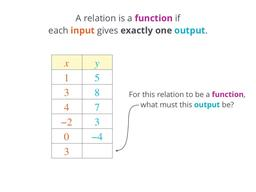FunctionsRelations where each input gives exactly one output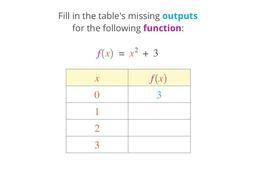Functional notationExplore what f(x) means, and evaluate functions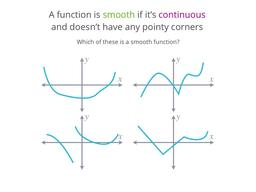Continuity and smoothnessSome functions end and others keep going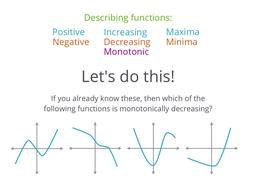Describing functionsMake your own functions, and live to tell the tale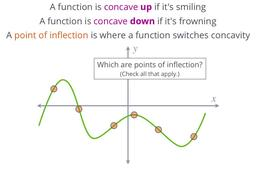ConcavityMath-talk for "curvy"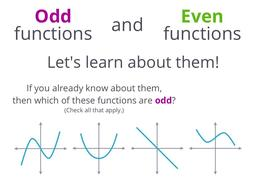Odd and even functionsTurns out functions can be "odd" and "even" too
4. Exponents
5. Logarithms
6. Playing with functions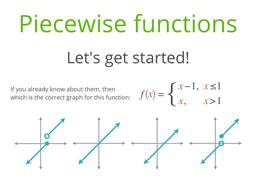Piecewise functionsFranken-functions. They're alive!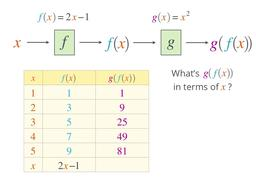CompositionsFunctions of functions
7. Conic sections
8. Rational functions
9. Polar functions